Home | | Maths 12th Std | Solved Example Problems on Inverse Trigonometric Functions

# Solved Example Problems on Inverse Trigonometric Functions

Mathematics : Inverse Trigonometric Functions: Solved Example Problems

Sine Function and Inverse Sine Function

### Example 4.1

Find the principal value of sin-1 ( - 1/2 ) (in radians and degrees).

### Solution

Let sin-1 ( - 1/2 ) = . Then sin = - 1/2 .

The range of the principal value of sin-1 is [- π/2 π/2 ] and hence, let us find  [- π/2 π/2 ] such that sin = - 1/2 . Clearly, y = - π /6.

Thus, the principal value of sin-1 ( - 1/2 ) is – π/6 . This corresponds to − 30°.

### Example 4.2

Find the principal value of sin-1 (2) , if it exists.

### Solution

Since the domain of = sin-1 is [-1, 1] and 2   [-1, 1] , sin-1 (2) does not exist.

### Example 4.3

Find the principal value of### Solution

We know that sin-1 : [-1, 1] → [- π/2 π/2] is given by

sin-1 if and only if = sin for -1 ≤ ≤ 1 and  π/2 ≤ y ≤ π/2 . Thus,Example 4.4

Find the domain of sin-1 (2 - 3x2)

### Solution

We know that the domain of sin-1 ( x) is [-1, 1].

This leads to -1 ≤ 2 - 3x2 ≤ 1, which implies - 3 ≤ -3x2 ≤ -1.

Now, - 3 ≤ -3x2 ,  gives x2 ≤ 1 and ... (1)

-3x2  ≤ -1 ,  gives x2  ≥ 1/3 ... (2)

Combining the equations (1) and (2), we get 1/3  ≤ x2 ≤ 1 . That is, 1/√3  ≤ |x| ≤ 1, which gives, since a ≤  |x| ≤ implies x ∈ [-b, - a]U[ab].

The Cosine Function and Inverse Cosine  Function

### Example 4.5

Find the principal value of cos-1 ( √3 / 2 ) .

### Solution

Let cos-1 (√3 / 2 ) = y . Then, cos y = √3 / 2.

The range of the principal values of = cos-1 is [0, π ].

So, let us find in [0, π ] such that cos y = √3 / 2

But, cos π/6 =  √3/2   and  π/6 ∈ [0,π ]. Therefore,  y = π /6

Thus, the principal value of cos-1 (√3/2 ) is π/6 .

### Example 4.6

Find### Solution

It is known that cos-1 : [-1, 1] → [0, π ] is given by

cos-1 if and only if = cos y for -1 ≤ ≤ 1 and 0 ≤ ≤ π .

Thus, we haveExample 4.7

Find the domain of cos-1 ( [2 + sin x] /3 ) .

Solution

By definition, the domain of = cos-1 x  is -1 ≤ ≤ 1 or |x| ≤1 . This leads to

-1 ≤  [2 + sin x]/≤ 1 which is same as - 3 ≤ 2 + sin ≤ 3 .

So, - 5 ≤ sin ≤ 1  reduces to -1 ≤ sin ≤ 1, which gives

- sin-1 (1) ≤ ≤ sin-1 (1) or  π/2 ≤ x ≤ π/2 .

Thus, the domain of cos-1 ( [2 + sin x] /3 )  is [- π/2 , π/2 ] .

The Tangent Function and the Inverse Tangent Function

Example 4.8

Find the principal value of tan-1 (√3)

Solution

Let tan-1 (√3) = y .

Then, tan y = √3.

Thus, y = π/3 . Since π/3 ∈ ( - π/2 , π/2 ) .

Thus, the principal value of tan-1(√3) is π/3.

### Example 4.9

Find (i) tan-1 (-√3 )

(ii) tan-1 ( tan (3π / 5))

(iii) tan (tan-1 (2019))

### Solution(iii) Since tan (tan-1  x) = x, x  R , we have   tan (tan-1 (2019)) = 2019.

### Example 4.10

Find the value of tan-1 (-1) + cos-1 ( 1/2 ) + sin-1 ( - 1/2 ) .

### Solution

Let tan-1 (-1) = y . Then, tan y = -1 = - tan π/4 = tan ( - π/4 ) .Example 4.11

Prove that   tan (sin-1 x) =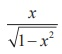, -1 < x < 1

### Solution

If = 0 , then both sides are equal to 0.           ………..(1)

Assume that 0 < < 1.

Let θ = sin-1 x . Then  0 < θ < π/2 .  Now, sin θ =  x/1  gives tanθ =.

Hence,   tan (sin-1x) =... (2)

Assume that -1 < x < 0. Then, θ = sin-1x gives – π/2  < θ < 0. Now, sinθ = x/1 gives tanθ =In this case also,  tan (sin-1 x) =... (3)

Equations (1), (2) and (3) establish that  tan (sin-1 x) =-1 < x < 1.

Principal Value of Inverse Trigonometric Functions

### Example 4.12

Find the principal value of

(i) cosec-1 (-1) (ii) sec-1 (-2) .

### Solution

(i) Let cosec-1 (-1) = . Then, cosec = -1

Since the range of principal value branch of y= cosec-1 x is [- π/2 , π/2] \ {0} andThus, the principal value of cosec-1 (-1) is – π/2 .

(ii) Let = sec-1 (-2) . Then, sec = -2 .

By definition, the range of the principal value branch of = sec-1 is [0,π ]\ {π /2} .

Let us find in [0,π ] – {π/2} such that sec = -2 .

But, sec y = −2  cos y = − 1/2 .

Now, cos y =- 1/2 = -cos π/3 = cos (π – π/3 ) = cos 2π/3 . Therefore, y = 2π/3 .

Since 2π/3 ∈ [0, π ] \ {π/2 } , the principal value of sec-1 (-2) is 2π/3 .

### Example 4.13

Find the value of sec-1(- 2√3 / 2)

### Solution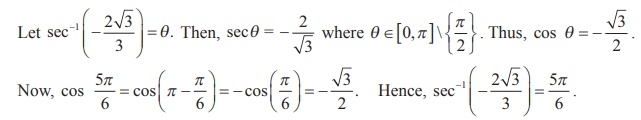### Example 4.14

If cot-1 ( 1/7 ) = θ , find the value of cos θ .

### Solution

By definition, cot−1 x  (0, π) .

Therefore, cot-1 (1/7) = θ implies cot θ ∈ (0,π ) .But cot-1 ( 1/7 ) = θ implies cot θ = 1/7 and hence tan θ = 7 and θ is acute.

Using tan θ = 7/1 , we construct a right triangle as shown . Then, we have, cosθ = 1/ 5√2  .

### Example 4.15

Show that> 1 .

### SolutionWe construct a right triangle with the given data.

From the triangle, secα = x/1 = x . Thus, α = sec-1 x .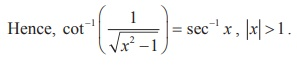OTHER

Example 4.16

Prove thatSolution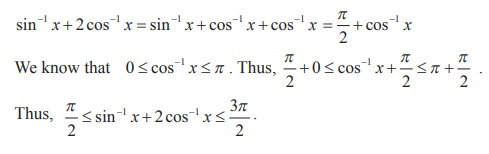Example 4.17Example 4.18SolutionExample 4.19Example 4.20Example 4.21Example 4.22

If cos-1 x + cos-1 y + cos-1 z = π and 0 < x, y, z < 1, show that

x2 + y2 + z2 + 2xyz = 1

Solution

Let cos-1 x = α and cos-1 y = β . Then, x = cosα  and  y = cos β .

cos-1 x + cos-1 y + cos-1 z = π gives α + β = π - cos-1 z.     ... (1)

Now, cos (α + β ) = cosα cos β - sin α sin β = xy – √[1-x2] √[1-y2]Squaring on both sides and simplifying, we get x2 + y2 + z2 + 2xyz = 1.

Example 4.23

If a1, a2, a3, ... an  is an arithmetic progression with common difference d, prove that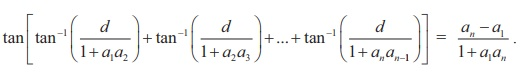SolutionExample 4.24Example 4.25

Solve sin-1 x > cos-1 x

Solution

Given that   sin-1 x > cos-1 x . Note that -1 ≤ x ≤ 1.

Adding both sides by sin-1 x, we get

sin-1 x + sin-1 x > cos-1 x + sin-1 x , which reduces to 2 sin-1 x > π/2 .

As sine function increases in the interval [- π/2 , π/2 ] , we have x > sin π/4 or x > 1/√2.

Thus, the solution set is the interval ( 1/√2, 1 ].

Example 4.26

Show that cot(sin-1 x) =, -1 ≤ x ≤ 1 and x ≠ 0

Solution

Let sin-1 x = θ . Then,  x = sin θ  and  x ± 0, we get θ [ -π/2, 0) U ( 0,       π/2].

Hence, cos θ ≥ 0 and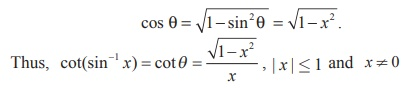Example 4.27

Solve tan-1 2x + tan-1 3x = π/4 , if 6x2 < 1.

Solution

Now, tan-1 2x + tan-13x = tan-1, since 6x2 < 1 .

So, tan-1=  π/4 , which implies 5x/ [1- 6x2] = tan π /4 = 1

Thus, 1- 6x2 = 5x , which gives 6x2 + 5x -1 = 0

Hence,         x = 1/6 , -1 . But x = -1 does not satisfy 6x2 < 1 .

Observe that         x = -1 makes the left side of the equation negative whereas the right side is a positive number. Thus, x = -1 is not a solution.

Hence, x = 1/6 is the only solution of the equation.

Example 4.28Example 4.29Tags : with Answers, Solution , 12th Mathematics : UNIT 4 : Inverse Trigonometric Functions
Study Material, Lecturing Notes, Assignment, Reference, Wiki description explanation, brief detail
12th Mathematics : UNIT 4 : Inverse Trigonometric Functions : Solved Example Problems on Inverse Trigonometric Functions | with Answers, Solution